Courses

# Electromagnetics - MCQ Test - 1

## 15 Questions MCQ Test GATE ECE (Electronics) 2022 Mock Test Series | Electromagnetics - MCQ Test - 1

Description
This mock test of Electromagnetics - MCQ Test - 1 for Electronics and Communication Engineering (ECE) helps you for every Electronics and Communication Engineering (ECE) entrance exam. This contains 15 Multiple Choice Questions for Electronics and Communication Engineering (ECE) Electromagnetics - MCQ Test - 1 (mcq) to study with solutions a complete question bank. The solved questions answers in this Electromagnetics - MCQ Test - 1 quiz give you a good mix of easy questions and tough questions. Electronics and Communication Engineering (ECE) students definitely take this Electromagnetics - MCQ Test - 1 exercise for a better result in the exam. You can find other Electromagnetics - MCQ Test - 1 extra questions, long questions & short questions for Electronics and Communication Engineering (ECE) on EduRev as well by searching above.
QUESTION: 1

Solution:
QUESTION: 2

### Stub matching is used to match the load to characteristic impedance. Stub should be placed such that the admittance value at that point is

Solution:

Stub matching is an effective technique in order to cancel out the reflectors when placed such that its reactive admittance value at the location of the stub is '0'. [Then it minimize losses]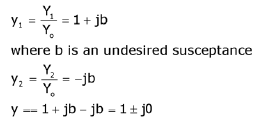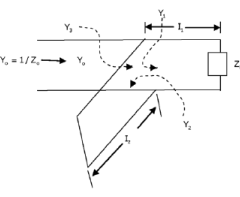QUESTION: 3

### Match the following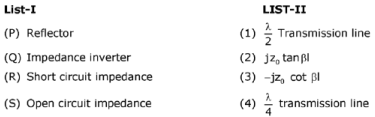Solution: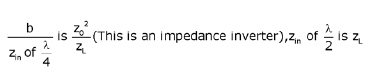QUESTION: 4

The characteristic impedance of a lossless transmission line at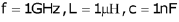is _________________ (ohms)

Solution: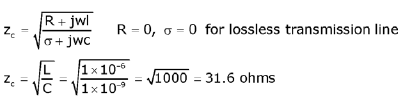QUESTION: 5

Assertion (A)    :    Magnetic field is continuous and always rotational.
Reason (R)    :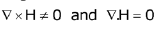Solution: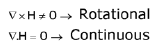So assertion and reason both are correct and reason is the correct explanation for assertion.

QUESTION: 6

An electric wave propagating through a lossless medium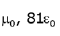is given by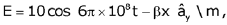:the phase constant β is given by

Solution: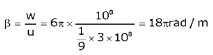QUESTION: 7

Assertion (A)    :    Polarization is always defined in terms of electric field orientation.
Reason (R)    :    Magnetic field is due to induced effect of electric field so we cannot measure it directly.

Solution:

Assertion and reason are correct and reason is the correct explanation for assertion

QUESTION: 8

Consider a wave which is having a phase velocity of 3x108m/s .Find the group ✓elocitv and the medium in which it is travelling

Solution: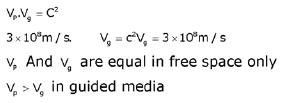QUESTION: 9

Phase difference between E and H in a high loss medium is _______________ (Degrees)

Solution: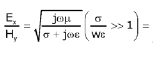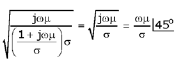QUESTION: 10

On the smith chart the location of the normalized load point is at 0.15λ, with reference to wavelength towards generator (WTG), scale operating wavelength is 4 cm. The location of first vmax from load is

Solution: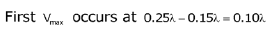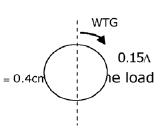QUESTION: 11

Which of the following statements on a smith chart are correct?
1.    Moving in clockwise direction is analogous to moving towards generator.
2.    VSWR circles can be plotted on a smith chart.
3.    Smith chart is the superposition of locus of VSWR and reflection coefficients.
4.    To complete a distance of 0.75λ. it's enough to move 0.5λ. towards aenerator.

Solution:

Smith chart is superposition of locus of resistance and reactance circles.

QUESTION: 12

S-matrix for two port transmission line is given by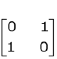. The reflection
coefficient and the transmission power through port 1 for input power of 5 mW is

Solution: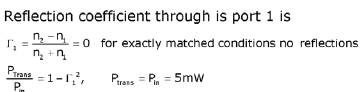QUESTION: 13

Match the following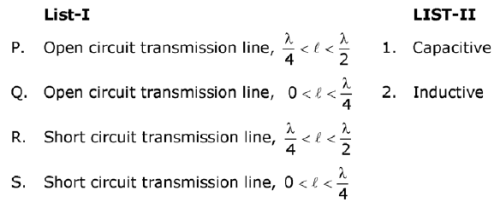Solution:
QUESTION: 14

For a waveguide component whose VSWR is 1.4 when the component is terminated with a matched load. The power to the matched load is 25 mW for an input power of 100 mW. The same results are obtained when component is reversed, then the S-matrix is

Solution: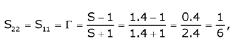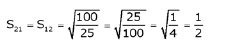QUESTION: 15

The propagation constant of a transmission line with impedance and admittance of 9 and 16 respectively is

Solution:

The propagation constant is given by γ = √(ZY), where Z is given by 9 and Y is 16. On substituting the given values, the propagation constant will be γ = √(ZY) = √(9 x 16) = 12 units.